Home | | Web Programming | Primitive Type Wrappers - Exploring java.lang

# Primitive Type Wrappers - Exploring java.lang

int and char, for performance reasons. These data types are not part of the object hierarchy.

Primitive Type Wrappers

As mentioned in Part I of this book, Java uses primitive types, such as int and char, for performance reasons. These data types are not part of the object hierarchy. They are passed by value to methods and cannot be directly passed by reference. Also, there is no way for two methods to refer to the same instance of an int. At times, you will need to create an object representation for one of these primitive types. For example, there are collection classes discussed in Chapter 18 that deal only with objects; to store a primitive type in one of these classes, you need to wrap the primitive type in a class. To address this need, Java provides classes that correspond to each of the primitive types. In essence, these classes encapsulate, or wrap, the primitive types within a class. Thus, they are commonly referred to as type wrappers. The type wrappers were introduced in Chapter 12. They are examined in detail here.

Number

The abstract class Number defines a superclass that is implemented by the classes that wrap the numeric types byte, short, int, long, float, and double. Number has abstract methods that return the value of the object in each of the different number formats. For example, doubleValue( ) returns the value as a double, floatValue( ) returns the value as a float, and so on. These methods are shown here:

byte byteValue( ) double doubleValue( ) float floatValue( )

int intValue( ) long longValue( ) short shortValue( )

The values returned by these methods might be rounded, truncated, or result in a “garbage” value due to the effects of a narrowing conversion.

Number has concrete subclasses that hold explicit values of each primitive numeric type: Double, Float, Byte, Short, Integer, and Long.

Double and Float

Double and Float are wrappers for floating-point values of type double and float, respectively. The constructors for Float are shown here:

Float(double num) Float(float num)

Float(String str) throws NumberFormatException

As you can see, Float objects can be constructed with values of type float or double. They can also be constructed from the string representation of a floating-point number.

The constructors for Double are shown here:

Double(double num)

Double(String str) throws NumberFormatException

Double objects can be constructed with a double value or a string containing a floating-point value.

The methods defined by Float include those shown in Table 17-1. The methods defined by Double include those shown in Table 17-2. Both Float and Double define the following constants: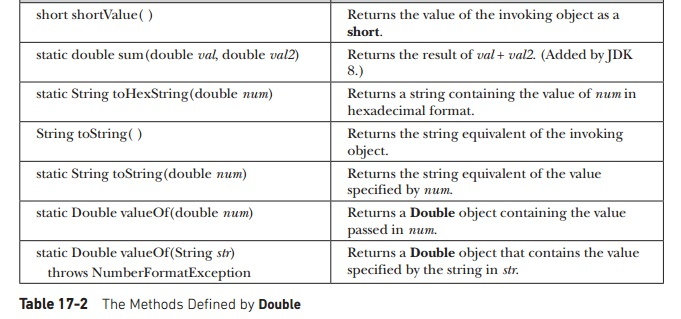The following example creates two Double objects—one by using a double value and the other by passing a string that can be parsed as a double:

class DoubleDemo {

public static void main(String args[]) { Double d1 = new Double(3.14159); Double d2 = new Double("314159E-5");

System.out.println(d1 + " = " + d2 + " -> " + d1.equals(d2));

}

}

As you can see from the following output, both constructors created identical Double instances, as shown by the equals( ) method returning true:

3.14159 = 3.14159 –> true

Understanding isInfinite( ) and isNaN( )

Float and Double provide the methods isInfinite( ) and isNaN( ), which help when manipulating two special double and float values. These methods test for two unique values defined by the IEEE floating-point specification: infinity and NaN (not a number). isInfinite( ) returns true if the value being tested is infinitely large or small in magnitude. isNaN( ) returns true if the value being tested is not a number.

The following example creates two Double objects; one is infinite, and the other is not a number:

// Demonstrate isInfinite() and isNaN()

class InfNaN {

public static void main(String args[]) { Double d1 = new Double(1/0.);

Double d2 = new Double(0/0.);

System.out.println(d1 + ": " + d1.isInfinite() + ", " + d1.isNaN()); System.out.println(d2 + ": " + d2.isInfinite() + ", " + d2.isNaN());

}

}

This program generates the following output:

Infinity: true, false

NaN: false, true

Byte, Short, Integer, and Long

The Byte, Short, Integer, and Long classes are wrappers for byte, short, int, and long integer types, respectively. Their constructors are shown here:

Byte(byte num)

Byte(String str) throws NumberFormatException

Short(short num)

Short(String str) throws NumberFormatException

Integer(int num)

Integer(String str) throws NumberFormatException

Long(long num)

Long(String str) throws NumberFormatException

As you can see, these objects can be constructed from numeric values or from strings that contain valid whole number values.

The methods defined by these classes are shown in Tables 17-3 through 17-6. As you can see, they define methods for parsing integers from strings and converting strings back into integers. Variants of these methods allow you to specify the radix, or numeric base, for conversion. Common radixes are 2 for binary, 8 for octal, 10 for decimal, and 16 for hexadecimal.

The following constants are defined: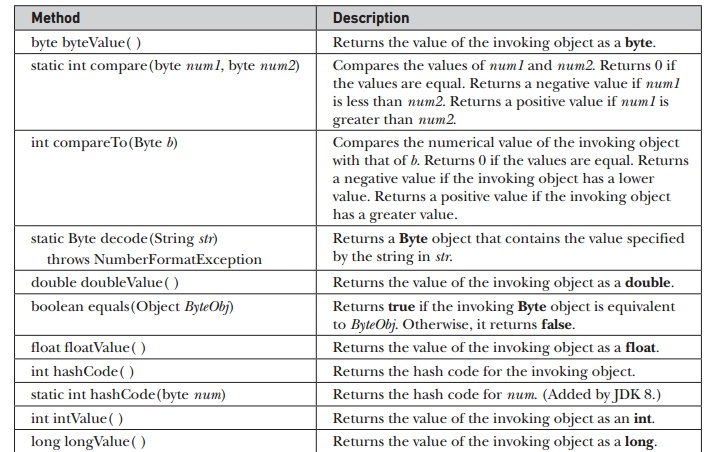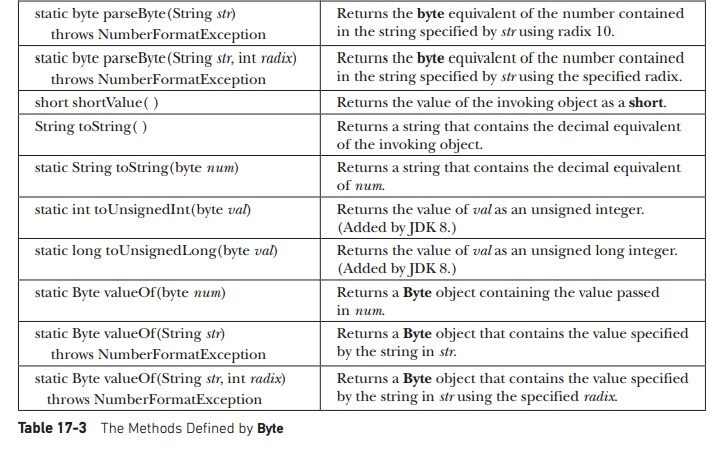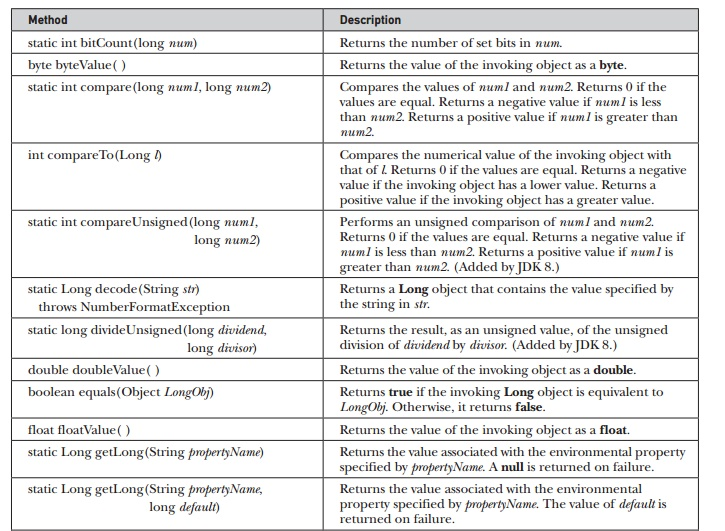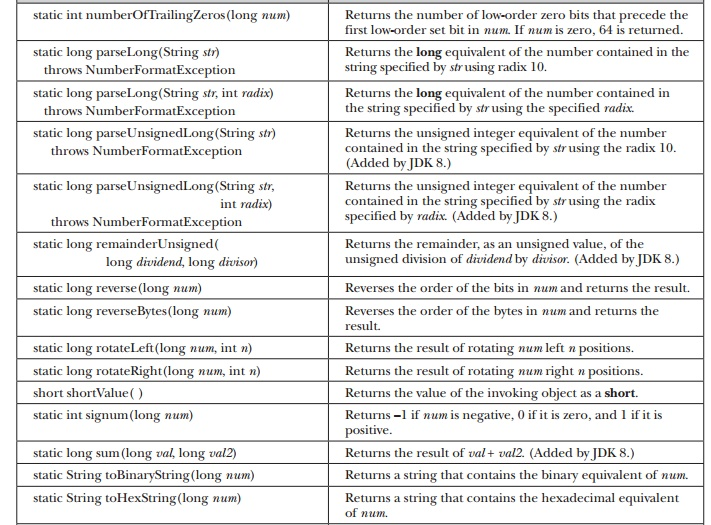Converting Numbers to and from Strings

One of the most common programming chores is converting the string representation of a number into its internal, binary format. Fortunately, Java provides an easy way to accomplish this. The Byte, Short, Integer, and Long classes provide the parseByte( ), parseShort( ), parseInt( ), and parseLong( ) methods, respectively. These methods return the byte, short, int, or long equivalent of the numeric string with which they are called. (Similar methods also exist for the Float and Double classes.)

The following program demonstrates parseInt( ). It sums a list of integers entered by the user. It reads the integers using readLine( ) and uses parseInt( ) to convert these strings into their int equivalents.

/* This program sums a list of numbers entered

by the user. It converts the string representation of each number into an int using parseInt().

*/

import java.io.*;

class ParseDemo {

public static void main(String args[]) throws IOException

{

int i; int sum=0;

System.out.println("Enter numbers, 0 to quit."); do {

i = Integer.parseInt(str);

} catch(NumberFormatException e) { System.out.println("Invalid format"); i = 0;

}

sum += i;

System.out.println("Current sum is: " + sum); } while(i != 0);

}

}

To convert a whole number into a decimal string, use the versions of toString( ) defined in the Byte, Short, Integer, or Long classes. The Integer and Long classes also provide the methods toBinaryString( ), toHexString( ), and toOctalString( ), which convert a value into a binary, hexadecimal, or octal string, respectively.

The following program demonstrates binary, hexadecimal, and octal conversion:

/*     Convert an integer into binary, hexadecimal, and octal.

*/

class StringConversions {

public static void main(String args[]) { int num = 19648;

System.out.println(num + " in binary: " + Integer.toBinaryString(num));

System.out.println(num + " in octal: " +

Integer.toOctalString(num));

System.out.println(num + " in hexadecimal: " + Integer.toHexString(num));

}

}

The output of this program is shown here:

19648 in binary: 100110011000000

19648 in octal: 46300

Character

Character is a simple wrapper around a char. The constructor for Character is Character(char ch)

Here, ch specifies the character that will be wrapped by the Character object being created. To obtain the char value contained in a Character object, call charValue( ), shown here:

char charValue( )

It returns the character.

The Character class defines several constants, including the following:Character includes several static methods that categorize characters and alter their case. A sampling is shown in Table 17-7. The following example demonstrates several of these methods:

// Demonstrate several Is... methods.

class IsDemo {

public static void main(String args[]) { char a[] = {'a', 'b', '5', '?', 'A', ' '};

for(int i=0; i<a.length; i++) { if(Character.isDigit(a[i]))

System.out.println(a[i] + " is a digit."); if(Character.isLetter(a[i]))

System.out.println(a[i] + " is a letter."); if(Character.isWhitespace(a[i]))

System.out.println(a[i] + " is whitespace."); if(Character.isUpperCase(a[i]))

System.out.println(a[i] + " is uppercase."); if(Character.isLowerCase(a[i]))

System.out.println(a[i] + " is lowercase.");

}

}

}

The output from this program is shown here:

a is    a letter.

a is    lowercase.

b is    a letter.

b is    lowercase.

5        is       a digit.

A is   a letter.

A is   uppercase.

is       whitespace.

Character defines two methods, forDigit( ) and digit( ), that enable you to convert between integer values and the digits they represent. They are shown here:

static char forDigit(int num, int radix) static int digit(char digit, int radix)

forDigit( ) returns the digit character associated with the value of num. The radix of the conversion is specified by radix. digit( ) returns the integer value associated with the specified character (which is presumably a digit) according to the specified radix. (There is a second form of digit( ) that takes a code point. See the following section for a discussion of code points.)

Another method defined by Character is compareTo( ), which has the following form: int compareTo(Character c)

It returns zero if the invoking object and c have the same value. It returns a negative value if the invoking object has a lower value. Otherwise, it returns a positive value.Character includes a method called getDirectionality( ) which can be used to determine the direction of a character. Several constants are defined that describe directionality. Most programs will not need to use character directionality.

Character also overrides the equals( ) and hashCode( ) methods.

Two other character-related classes are Character.Subset, used to describe a subset of Unicode, and Character.UnicodeBlock, which contains Unicode character blocks.

Additions to Character for Unicode Code Point Support

Relatively recently, major additions were made to Character. Beginning with JDK 5, the Character class has included support for 32-bit Unicode characters. In the past, all Unicode characters could be held by 16 bits, which is the size of a char (and the size of the value encapsulated within a Character), because those values ranged from 0 to FFFF. However, the Unicode character set has been expanded, and more than 16 bits are required. Characters can now range from 0 to 10FFFF.

Here are three important terms. A code point is a character in the range 0 to 10FFFF. Characters that have values greater than FFFF are called supplemental characters. The basic multilingual plane (BMP) are those characters between 0 and FFFF.

The expansion of the Unicode character set caused a fundamental problem for Java. Because a supplemental character has a value greater than a char can hold, some means of handling the supplemental characters was needed. Java addressed this problem in two ways. First, Java uses two chars to represent a supplemental character. The first char is called the high surrogate, and the second is called the low surrogate. New methods, such as codePointAt( ), were provided to translate between code points and supplemental characters.

Secondly, Java overloaded several preexisting methods in the Character class. The overloaded forms use int rather than char data. Because an int is large enough to hold any character as a single value, it can be used to store any character. For example, all of the methods in Table 17-7 have overloaded forms that operate on int. Here is a sampling:

static boolean isDigit(int cp) static boolean isLetter(int cp) static int toLowerCase(int cp)

In addition to the methods overloaded to accept code points, Character adds methods that provide additional support for code points. A sampling is shown in Table 17-8.

Boolean

Boolean is a very thin wrapper around boolean values, which is useful mostly when you want to pass a boolean variable by reference. It contains the constants TRUE and FALSE, which define true and false Boolean objects. Boolean also defines the TYPE field, which is the Class object for boolean. Boolean defines these constructors:

Boolean(boolean boolValue)

Boolean(String boolString)In the first version, boolValue must be either true or false. In the second version, if boolString contains the string "true" (in uppercase or lowercase), then the new Boolean object will be true. Otherwise, it will be false.

Boolean defines the methods shown in Table 17-9.Study Material, Lecturing Notes, Assignment, Reference, Wiki description explanation, brief detail
Java The Complete Reference : The Java Library : Exploring java.lang : Primitive Type Wrappers - Exploring java.lang |